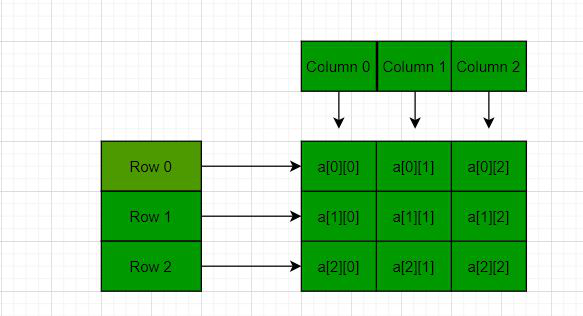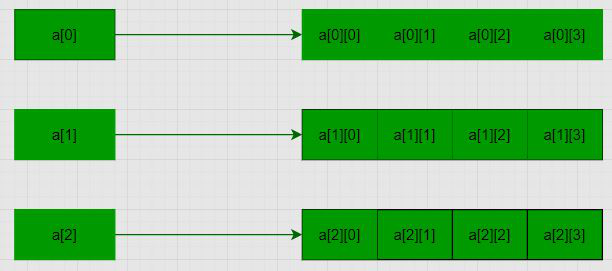# How to declare a 2D array dynamically in C++ using new operator

• Difficulty Level : Medium
• Last Updated : 14 Sep, 2022

Prerequisite: Array Basics
In C/C++, multidimensional arrays in simple words as an array of arrays. Data in multidimensional arrays are stored in tabular form (in row major order). Below is the general form of declaring N-dimensional arrays:

Syntax of a Multidimensional Array:

data_type array_name[size1][size2]….[sizeN];

data_type: Type of data to be stored in the array.
Here data_type is valid C/C++ data type
array_name: Name of the array
size1, size2, …, sizeN: Sizes of the dimensions

2D arrays are arrays of single-dimensional arrays.

Syntax of a 2D array:

data_type array_name[x][y];
data_type: Type of data to be stored. Valid C/C++ data type.

Below is the diagrammatic representation of 2D arrays:For more details on multidimensional and 2D arrays, please refer to Multidimensional arrays in C++ article.

Problem: Given a 2D array, the task is to dynamically allocate memory for a 2D array using new in C++.

Solution: Following 2D array is declared with 3 rows and 4 columns with the following values:

```1 2 3 4
5 6 7 8
9 10 11 12```

Note: Here M is the number of rows and N is the number of columns.

Method 1: using a single pointer – In this method, a memory block of size M*N is allocated and then the memory blocks are accessed using pointer arithmetic. Below is the program for the same:

## C++

 `// C++ program to dynamically allocate``// the memory for 2D array in C++``// using new operator``#include ``using` `namespace` `std;` `// Driver Code``int` `main()``{``    ``// Dimensions of the 2D array``    ``int` `m = 3, n = 4, c = 0;` `    ``// Declare a memory block of``    ``// size m*n``    ``int``* arr = ``new` `int``[m * n];` `    ``// Traverse the 2D array``    ``for` `(``int` `i = 0; i < m; i++) {``        ``for` `(``int` `j = 0; j < n; j++) {` `            ``// Assign values to``            ``// the memory block``            ``*(arr + i * n + j) = ++c;``        ``}``    ``}` `    ``// Traverse the 2D array``    ``for` `(``int` `i = 0; i < m; i++) {``        ``for` `(``int` `j = 0; j < n; j++) {` `            ``// Print values of the``            ``// memory block``            ``cout << *(arr + i * n + j)``                 ``<< ``" "``;``        ``}``        ``cout << endl;``    ``}``  ` `      ``//Delete the array created``      ``delete``[] arr;` `    ``return` `0;``}`

Output:

```1 2 3 4
5 6 7 8
9 10 11 12```

Method 2: using an array of pointer: Here an array of pointers is created and then to each memory block. Below is the diagram to illustrate the concept:Below is the program for the same:

## C++

 `// C++ program to dynamically allocate``// the memory for 2D array in C++``// using new operator``#include ``using` `namespace` `std;` `// Driver Code``int` `main()``{``    ``// Dimensions of the array``    ``int` `m = 3, n = 4, c = 0;` `    ``// Declare memory block of size M``    ``int``** a = ``new` `int``*[m];` `    ``for` `(``int` `i = 0; i < m; i++) {` `        ``// Declare a memory block``        ``// of size n``        ``a[i] = ``new` `int``[n];``    ``}` `    ``// Traverse the 2D array``    ``for` `(``int` `i = 0; i < m; i++) {``        ``for` `(``int` `j = 0; j < n; j++) {` `            ``// Assign values to the``            ``// memory blocks created``            ``a[i][j] = ++c;``        ``}``    ``}` `    ``// Traverse the 2D array``    ``for` `(``int` `i = 0; i < m; i++) {``        ``for` `(``int` `j = 0; j < n; j++) {` `            ``// Print the values of``            ``// memory blocks created``            ``cout << a[i][j] << ``" "``;``        ``}``        ``cout << endl;``    ``}` `    ``// Delete the array created``    ``for` `(``int` `i = 0; i < m; i++) ``// To delete the inner``                                ``// arrays``        ``delete``[] a[i];``    ``delete``[] a; ``// To delete the outer array``                ``// which contained the pointers``                ``// of all the inner arrays` `    ``return` `0;``}`

Output:

```1 2 3 4
5 6 7 8
9 10 11 12```

My Personal Notes arrow_drop_up